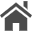Home  Updated: November 15, 2020  [日本語 / English]

# Measuring temperature using a Pt100 sensor (platinum resistance thermometer)

## Introduction

I used Pt100 sensor to control the temperature of the nozzle of my 3D printer. A resistance thermometer is a temperature sensor that utilizes the characteristics of metal whose resistance value changes with temperature. Pt100 is a platinum resistance thermometer with a resistance value of $$100 {\rm \Omega}$$ at $$0^\circ {\rm C}$$. The higher the temperature, the greater the resistance.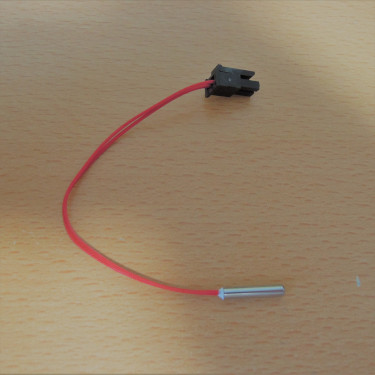## Resistance of Pt100

The resistance $$R_t [\Omega]$$ of Pt100 at temperature $$t [ ^\circ \rm C]$$ is expressed in the following equation. Where $$t \geqq 0$$. $$R_t = R_0 (1 + A t + B t^2)$$ $$A = 3.9083 \times 10^{-3}$$ $$B = -5.775 \times 10^{-7}$$ $$R_0 = 100 [\Omega]$$

If a graph is drawn, it looks like the figure below.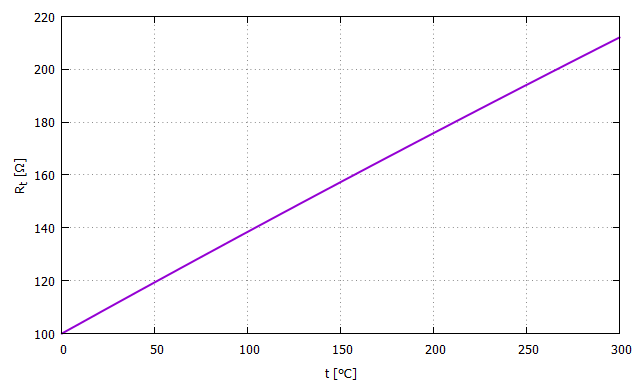## Measurement Circuit

In order to convert the resistance of Pt100 to a voltage and input it to the microcontroller's AD converter, I built a circuit as shown below. Connect Pt100 to the connector J1.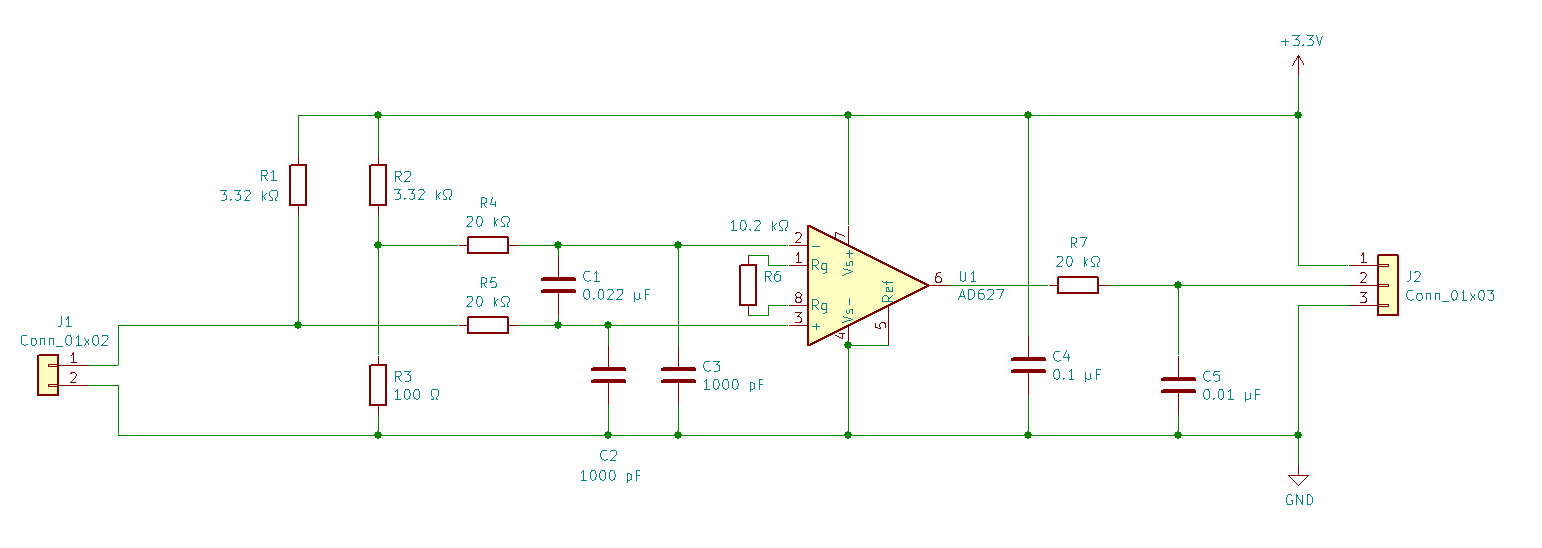First, the bridge is configured with the resistance $$R_t$$ of Pt100 and $$R_1$$, $$R_2$$, and $$R_3$$, and the voltage $$V_1$$ is obtained according to the resistance $$R_t$$. The output voltage of the bridge, $$V_1 [\rm V]$$, is expressed in the following equation $$V_1 = 3.3[{\rm V}] \times \left( \frac{R_t}{R_1 + R_t} - \frac{R_3}{R_2 + R_3} \right)$$

$$R_4$$, $$R_5$$, $$C_1$$, $$C_2$$ and $$C_3$$ constitute the low-pass filter.

Next, the AD627 instrumentation amplifier is used to amplify it by about 25 times to bring it into a range that can be easily handled by an AD converter. The relationship between the output voltage $$V_2 [\rm V]$$ of the instrumentation amplifier and temperature $$t [ ^\circ \rm C]$$ is shown in the figure below.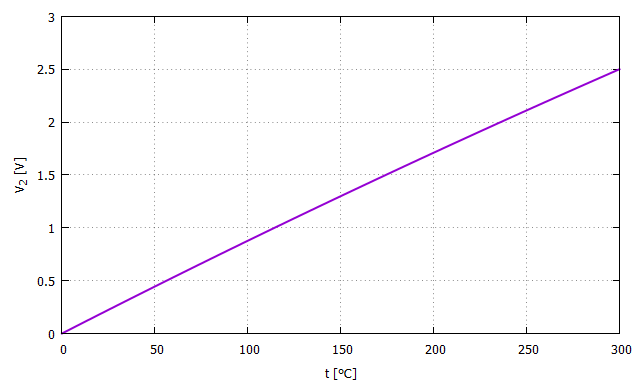This relationship can be used to calculate $$t$$ backwards from the AD conversion result.

## Commercially available Pt100 sensors

omron E52 Temperature Sensor (General Purpose Type)